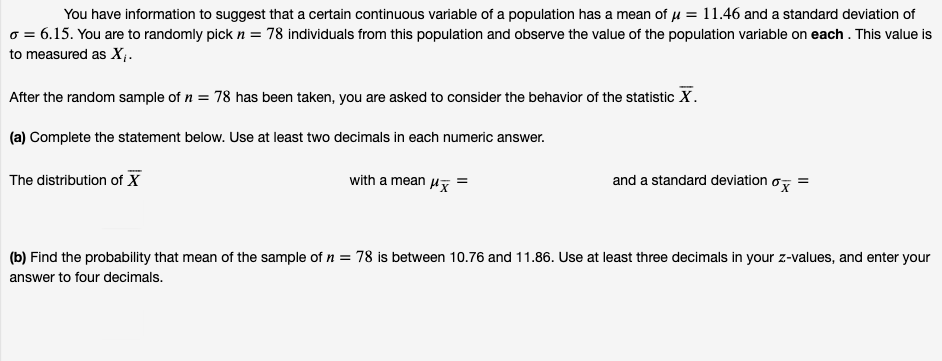# You have information to suggest that a certain continuous variable of a populaton has a mean of μ-11.46 and a standard deva ton ofσ = 6.15. You are to randomly pick n = 78 individuals from this population and observe the value of the population variable on each . This valuesto measured as XAfter the random sample of n = 78 has been taken, you are asked to consider the behavior of the statistic X(a) Complete the statement below. Use at least two decimals in each numeric answer.The distribution of Xwith a mean μ5--and a standard deviation σ(b) Find the probability that mean of the sample of n = 78 is between 10.76 and 1 1.86. Use at least three decimals in your z-values, and enter youranswer to four decimals.

Questionhelp_outlineImage TranscriptioncloseYou have information to suggest that a certain continuous variable of a populaton has a mean of μ-11.46 and a standard deva ton of σ = 6.15. You are to randomly pick n = 78 individuals from this population and observe the value of the population variable on each . This values to measured as X After the random sample of n = 78 has been taken, you are asked to consider the behavior of the statistic X (a) Complete the statement below. Use at least two decimals in each numeric answer. The distribution of X with a mean μ5-- and a standard deviation σ (b) Find the probability that mean of the sample of n = 78 is between 10.76 and 1 1.86. Use at least three decimals in your z-values, and enter your answer to four decimals. fullscreen
check_circle

Step 1

Given that a continuous variable of a population with mean = 11.46 and standard deviation = 6.15.

A sample of n = 78 is randomly picked

According to central limit theorem From any population with mean µ and standard deviation σ, random samples of large size (n) are chosen, then the distribution of sample means Xbar will be normal distribution with mean µ and standard deviation σ/√n.

So the distribution of Xbar (sample means) will be normal distribution with sample size       n = 78  and the sample mean = 11.46 and sample standard deviation = 0.70 (rounded to two decimals) are shown below.

Step 2

We need to find the probability that mean of the sample of n = 78 is between 10.76 and 11.86.

So we need P( 10.76 < Xbar < 11.86...

### Want to see the full answer?

See Solution

#### Want to see this answer and more?

Solutions are written by subject experts who are available 24/7. Questions are typically answered within 1 hour.*

See Solution
*Response times may vary by subject and question.
Tagged in

### Statistics